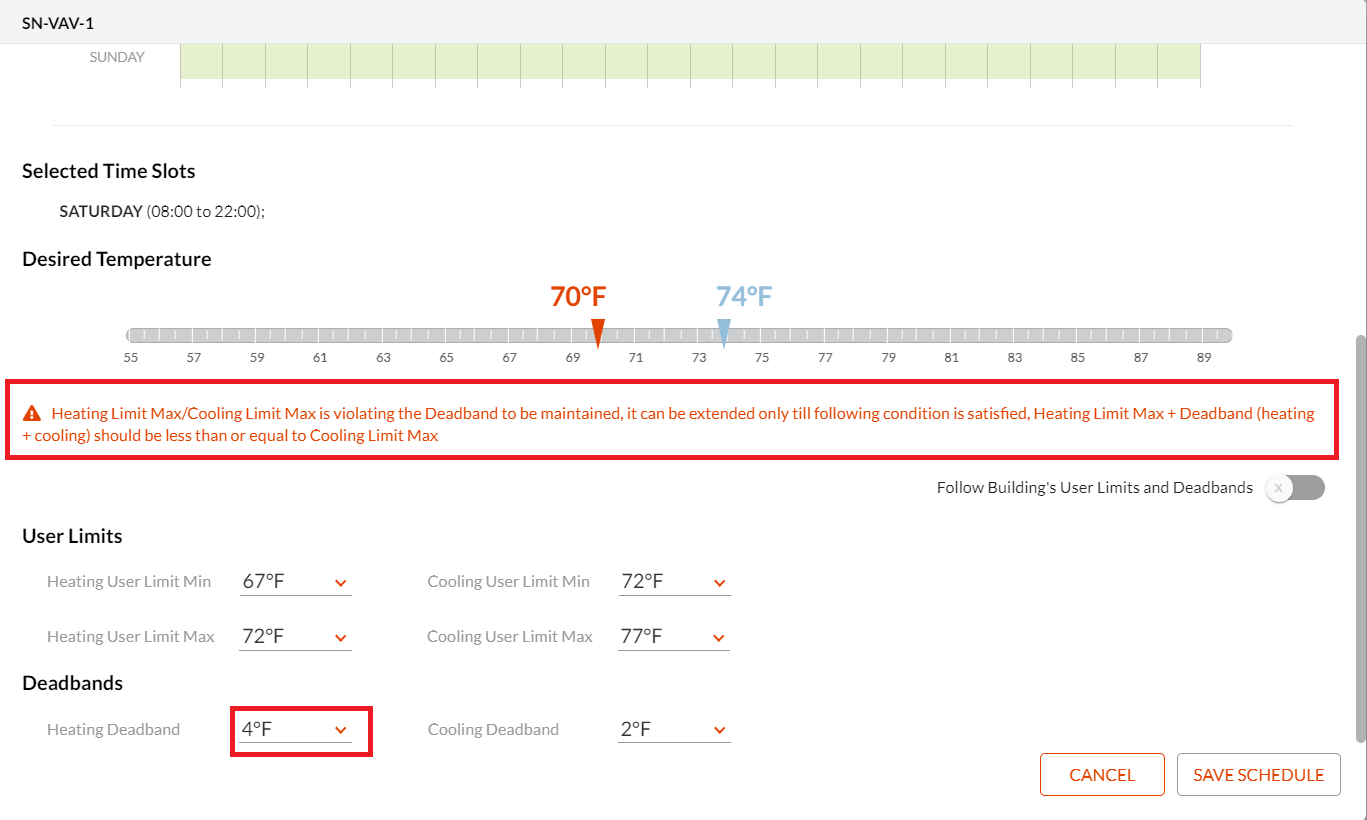## browse

### | Introduction

Scheduler validations are a set of rules that need to be satisfied for the scheduler to perform without any consistent errors or anomalies so as to get the desired outputs from a schedule.

### | Validations

1. Building Occupancy Overall Containment

(a) Building Occupancy Overall Containment for Zone Schedule

The start and stop time for a zone schedule should be within the defined time of the building occupancy.

Let us consider an example of a zone schedule where the start and stop time defined for a building occupancy is 8.00 a.m. and 10 p.m. respectively. If the user sets the time to 10:30 p.m., then the scheduler will display an error.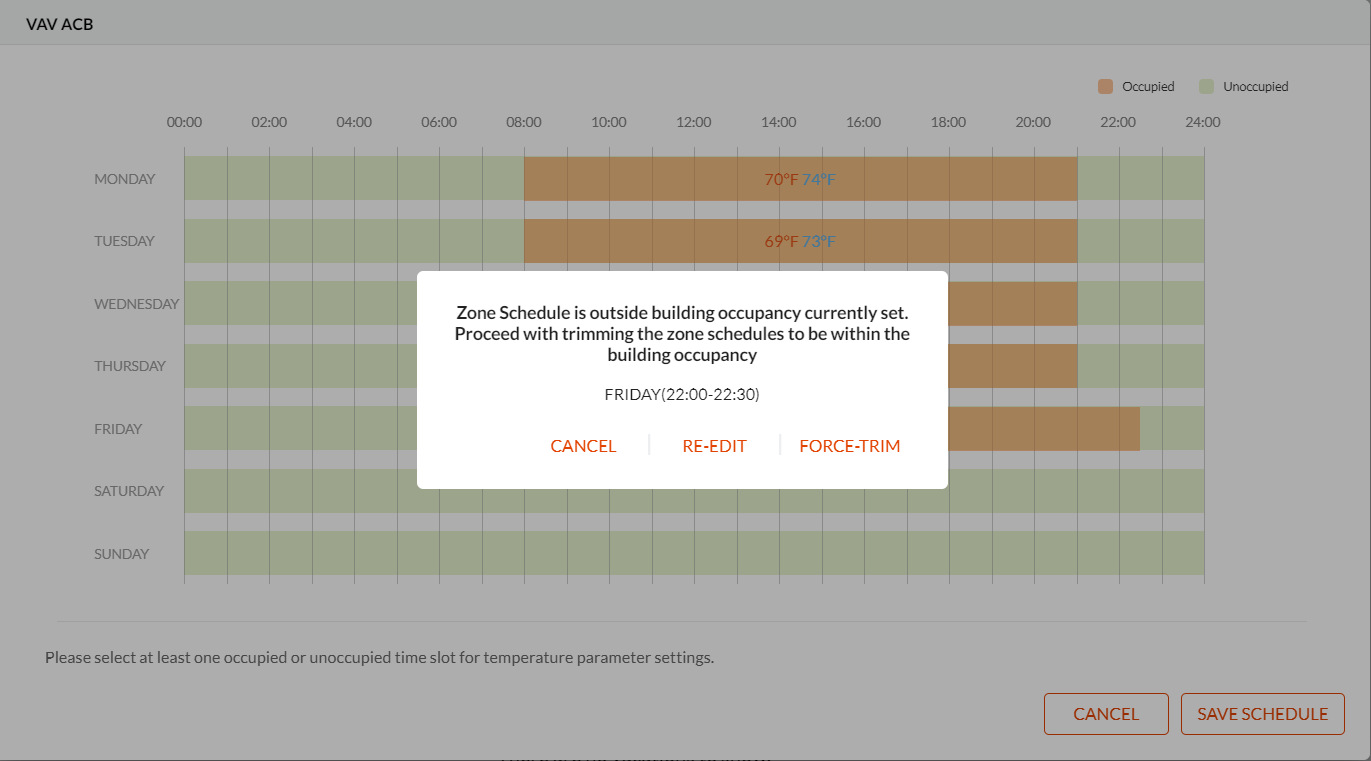(b) Building Occupancy Overall Containment for Named Schedule

The start and stop time for a named schedule should be within the defined time of the building occupancy.

Let us consider an example of a named schedule where the start and stop time defined for a building occupancy is 8.00 a.m. and 10.00 p.m. respectively. If the user sets the time to 10:30 p.m., then the scheduler will display an error.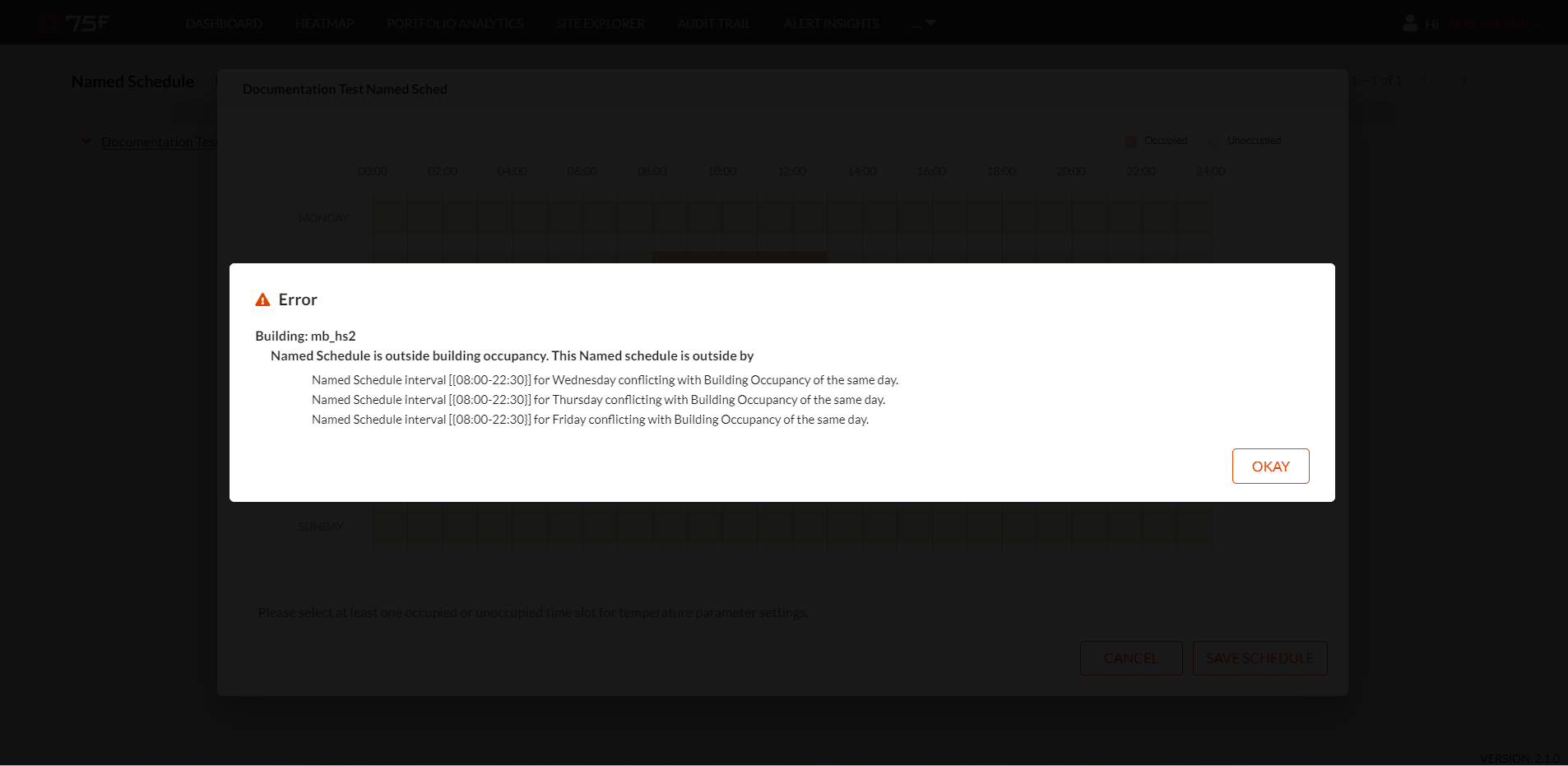2. User Limits within Building Limits (For Both Heating and Cooling)

The user limits defined for a schedule should be within the defined building limits.

Let us consider an example of a schedule where the user limits defined for heating and cooling are 67F and 72F and 72F and 77F respectively. If the user tries to set the schedule beyond the defined building limits i.e. (55F and 90F in the default case), then it will display an error.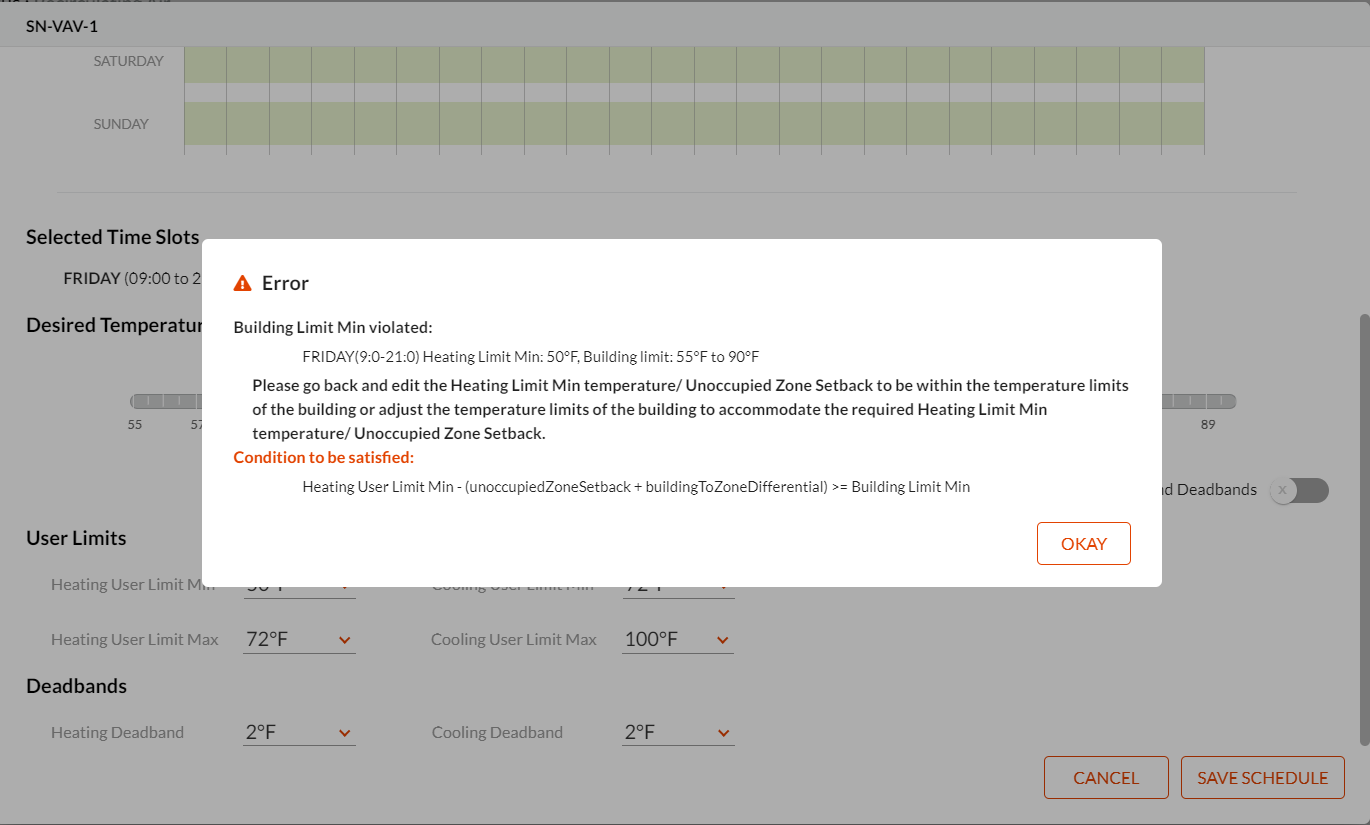3. Impact of the Sum of Building Zone Differential and Unoccupied Setback

(a)The first validation deals with the case when the minimum heating user limit should not fall behind the total of (Minimum Building limit + (Sum of Building Zone Differential and Unoccupied Setback))

Let us consider an example of a building where the minimum building limit is 55F, and the minimum heating user limit is 67F. In this case, the user limit should not fall behind 63F or else it will display an error message indicating an anomaly.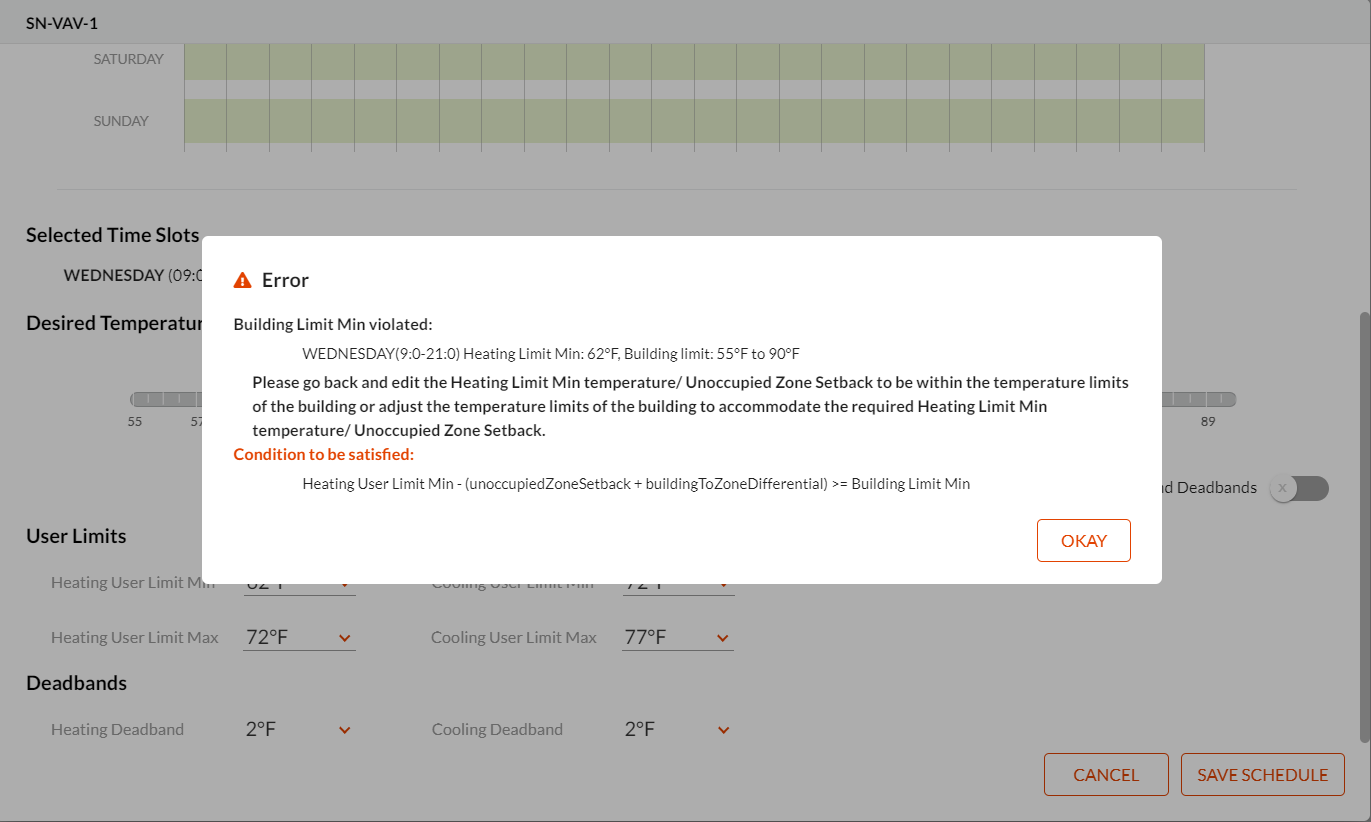(b) The second validation deals with the case where the maximum cooling user limit should not exceed the total of (Maximum Building limit - (Sum of Building Zone Differential and Unoccupied Setback))

Let us consider an example of a building where the maximum building limit is 90F, and the maximum cooling user limit is 77F. In this case, the user limit should not exceed 82F or else it will display an error message indicating an anomaly.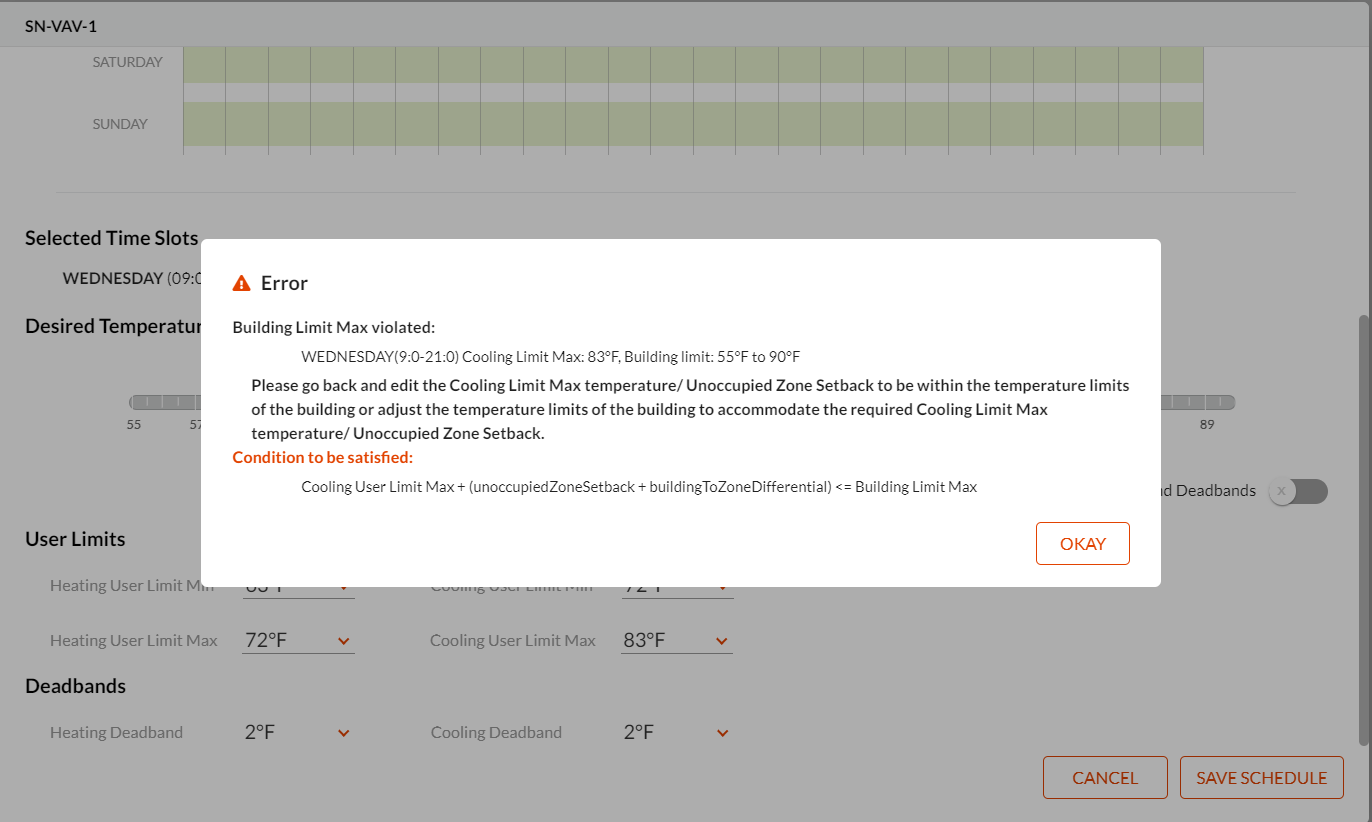4. Overlapping User Limits

User limits in a schedule can only overlap each other when:

(a) Maximum Heating User limit + (Heating Deadband+Cooling Deadband) <= Maximum Cooling User limit

Let us consider an example where the maximum heating user limit for a schedule is 72F, the heating and cooling deadband is 2F and 2F respectively and the maximum cooling user limit is 77F.

In this case,

72F + (2F+2F) <= 77F or else the scheduler will display an error as follows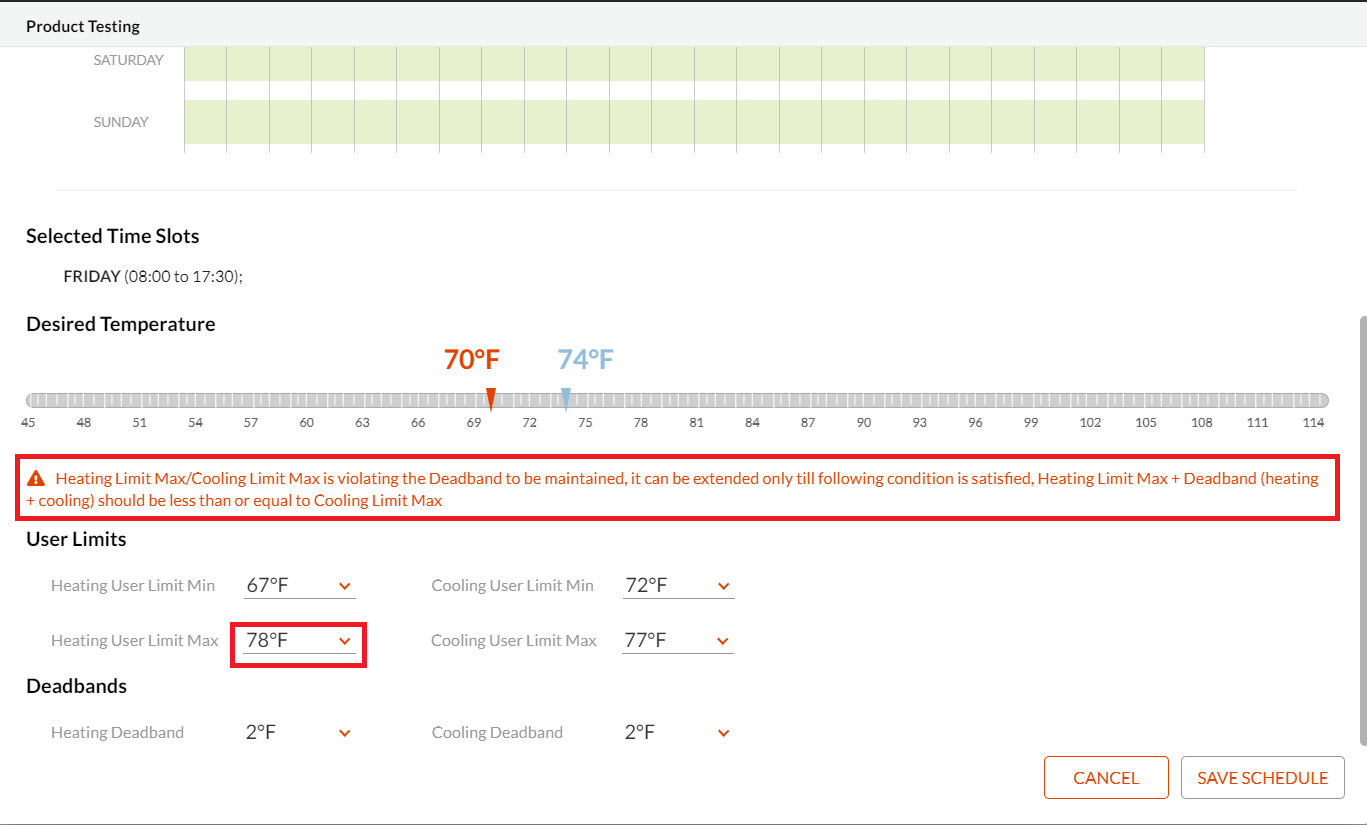(b) Minimum Cooling Limit - (Heating Deadband+ Cooling Deadband) >= Minimum Heating User Limit

Let us consider an example where the minimum cooling user limit for a schedule is 70F, the heating and cooling deadband is 2F and 2F respectively and the minimum heating user limit is 66F.

In this case,

70F - (2F+2F) >= 66F or else the scheduler will display an error as follows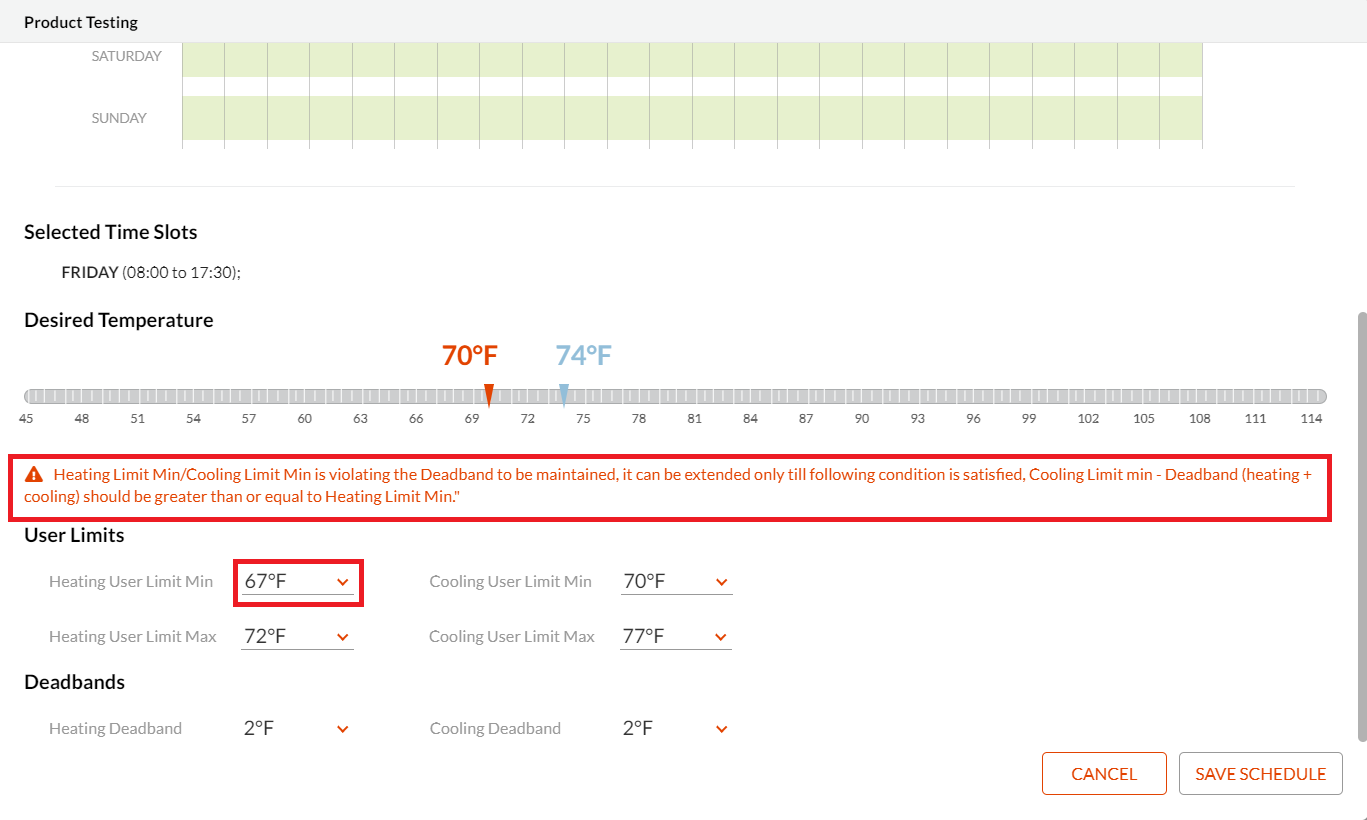5. Desired Temperatures should always be separated by Deadbands

Modifying the heating or cooling deadband for a schedule will result in an error where the heating desired temperature and cooling desired temperature in a schedule are defined since a minimum difference has to be maintained for the desired temperatures to take effect.

Let us consider an example where the heating desired temperature is defined as 70F, and the cooling desired temperature is defined as 74F along with a heating and cooling deadband of 2F respectively.

If we try to increase or decrease the defined deadbands then it will result in an error as shown below: